## Elliptic Curve Group Law

The Group of an Elliptic Curve which has been transformed to the form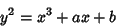is the set of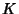-Rational Points, including the single Point at Infinity. The group law (addition) is defined as follows: Take 2-Rational Pointsand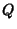. Now `draw' a straight line through them and compute the third point of intersection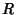(also a-Rational Point). Thengives the identity point at infinity. Now find the inverse of, which can be done by setting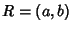giving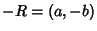.

This remarkable result is only a special case of a more general procedure. Essentially, the reason is that this type of Elliptic Curve has a single point at infinity which is an inflection point (the line at infinity meets the curve at a single point at infinity, so it must be an intersection of multiplicity three).